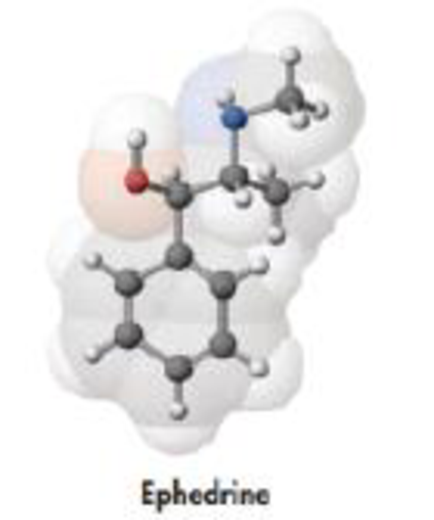Chapter 2, Problem 128GQ

Chapter
Section
Textbook Problem

Ma huang, an extract from the ephedra species of plants, contains ephedrine. The Chinese have used this herb for more than 5000 years to treat asthma. More recently, ephedrine has been used in diet pills that can be purchased over the counter in herbal medicine shops. However, very serious concerns have been raised regarding these pills following reports that their use led to serious heart problems.(a) A molecular model of ephedrine is drawn below. From this determine the molecular formula for ephedrine and calculate its molar mass.(b) What is the weight percent of carbon in ephedrine?(c) Calculate the amount (moles) of ephedrine in a 0.125 g sample.(d) How many molecules of ephedrine are there in 0.125 g? How many C atoms?(a)

Interpretation Introduction

Interpretation: The molecular formula for ephedrine and its molar mass should be determined.

Concept introduction:

• Moleofsubstance=GramofsubstanceMolarmass
• A chemical formula conveys us the number of atoms of each element in a compound. It contains the symbols of the atoms of the elements present in the compound as well as how many are for each element in the form of subscripts
• The molar mass of an element or compound is the mass in grams of 1 mole of that substance, and it is expressed in the unit of grams per mol (g/mol)
• Weight percentage is one way to represent the concentration of an element in a compound or a component in a mixture. Weight percent of elements of a compound is the ratio of weight of element to the weight of whole compound and multiplied with hundred.
Explanation

Given,

The molecular model of ephedrine is,

There are ten carbon atoms, one oxygen atoms fifteen hydrogen atoms and one nitrogen atom

Therefore molecular formula of ephedrine is C10H15NO

Molar mass of ephedrine can be calculated as follows,

(10×12.01massofC)+(15×1massof

(b)

Interpretation Introduction

Interpretation: The weight for carbon present in ephedrine should be determined.

Concept introduction:

• Moleofsubstance=GramofsubstanceMolarmass
• A chemical formula conveys us the number of atoms of each element in a compound. It contains the symbols of the atoms of the elements present in the compound as well as how many are for each element in the form of subscripts
• The molar mass of an element or compound is the mass in grams of 1 mole of that substance, and it is expressed in the unit of grams per mol (g/mol)
• Weight percentage is one way to represent the concentration of an element in a compound or a component in a mixture. Weight percent of elements of a compound is the ratio of weight of element to the weight of whole compound and multiplied with hundred.

(c)

Interpretation Introduction

Interpretation: The amount of ephedrine present in 0.125gsample of given ephedrine compound should be determined.

Concept introduction:

• Moleofsubstance=GramofsubstanceMolarmass
• A chemical formula conveys us the number of atoms of each element in a compound. It contains the symbols of the atoms of the elements present in the compound as well as how many are for each element in the form of subscripts
• The molar mass of an element or compound is the mass in grams of 1 mole of that substance, and it is expressed in the unit of grams per mol (g/mol)
• Weight percentage is one way to represent the concentration of an element in a compound or a component in a mixture. Weight percent of elements of a compound is the ratio of weight of element to the weight of whole compound and multiplied with hundred.

(d)

Interpretation Introduction

Interpretation: The molecules of ephedrine present in 0.125gsample of given ephedrine compound and the number of carbon atoms should be determined.

Concept introduction:

• Moleofsubstance=GramofsubstanceMolarmass
• A chemical formula conveys us the number of atoms of each element in a compound. It contains the symbols of the atoms of the elements present in the compound as well as how many are for each element in the form of subscripts
• The molar mass of an element or compound is the mass in grams of 1 mole of that substance, and it is expressed in the unit of grams per mol (g/mol)
• Weight percentage is one way to represent the concentration of an element in a compound or a component in a mixture. Weight percent of elements of a compound is the ratio of weight of element to the weight of whole compound and multiplied with hundred.

Still sussing out bartleby?

Check out a sample textbook solution.

See a sample solution

The Solution to Your Study Problems

Bartleby provides explanations to thousands of textbook problems written by our experts, many with advanced degrees!

Get Started

Name the following compounds. a Na2SO4 b Na3N c CuCl d Cr2O3

General Chemistry - Standalone book (MindTap Course List)

4. The normal arterial range is

CARDIOPULMONARY ANATOMY+PHYSIOLOGY

What impact has recombinant DNA technology had on genetics and society?

Human Heredity: Principles and Issues (MindTap Course List)